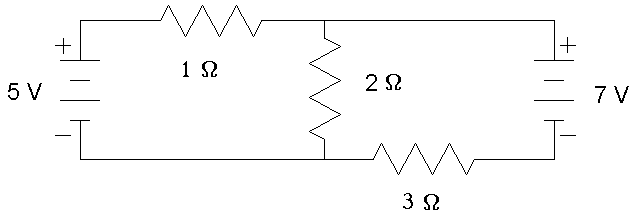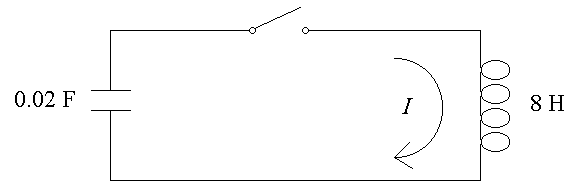First Name: ___________________ Last Name: ____________________ Section: _________

May 11, 1997 Physics 208

Final Exam

Print your name and section clearly on all nine pages. (If you do not know your section number, write your TAs name.) Show all work in the space immediately below each problem. Your final answer must be placed in the box provided. Problems will be graded on reasoning and intermediate steps as well as on the final answer. Be sure to include units wherever necessary, and the direction of vectors. Each problem is worth 25 points. In doing the problems, try to be neat. Check your answers to see that they have the correct dimensions (units) and are the right order of magnitudes. You are allowed two 8½ x 11" sheet of notes and no other references. The exam lasts exactly 2 hours.

(Do not write below)

SCORE:

Problem 1: __________

Problem 2: __________

Problem 3: __________

Problem 4: __________

Problem 5: __________

Problem 6: __________

Problem 7: __________

Problem 8: __________

TOTAL: ___________

First Name: ___________________ Last Name: ____________________ Section: _________

1. The nucleus of a gold atom (Z = 79) has a radius of 6.2 x 10-15 m and a charge which you may consider to be uniformly distributed throughout the nucleus.

a. What is the density of electric charge in the nucleus? (5 pts.)

b. What is the electric field strength at the surface of the nucleus? (5 pts.)

c. How much energy (in eV) is required to bring a proton from infinity to the surface of the nucleus? (5 pts.)

d. What is the capacitance of the nucleus relative to infinity? (5 pts.)

e. If this isotope of gold has A = 197, what is its approximate mass? (5 pts.)

First Name: ___________________ Last Name: ____________________ Section: _________

1. In the circuit below, calculate the voltage across the 2-W resistor. (25 pts.)
2.First Name: ___________________ Last Name: ____________________ Section: _________

3. A long, thin, straight beam consists of 1010 protons per centimeter of length, each moving with a speed of 5 x 106 m/s.
4.

a. What is the electric current carried by the beam? (5 pts.)

b. What is the magnetic field outside the beam, 30 cm from its center? (5 pts.)

c. What is the magnetic force on an electron (me = 9.1 x 10-31 kg), 30 cm from the center of the beam and moving with a velocity 1% the speed of light parallel to the beam? (5 pts.)

d. What is the radius of curvature of the orbit of the electron in part c above? (5 pts.)

e. What is the kinetic energy (in eV) of the electron in part c above? (5 pts.)

First Name: ___________________ Last Name: ____________________ Section: _________

5. circuit below is initially charged to 80 V, and the switch is closed at t = 0.
6.a. At what frequency f does the current I oscillate? (5 pts.)

b. What is the current I just after the switch closes? (5 pts.)

c. At what time after the switch closes does the current I first reach its maximum value? (5 pts.)

d. What is the maximum value of the current I? (5 pts.)

e. What is the current I at t = 0.4 s? (5 pts.)

First Name: ___________________ Last Name: ____________________ Section: _________

7. An electromagnetic wave with f = 5.5 x 1014 Hz traveling through the air is incident on a medium with an index of refraction of n = 1.5 at an angle of 40 degrees.
8. a. What is the wavelength of the wave in the air? (5 pts.)

b. What is the wavelength of the wave in the medium? (5 pts.)

c. What is the angle of the refracted wave in the medium? (5 pts.)

d. What is the angle of the reflected wave in the air? (5 pts.)

e. What is the phase shift of the reflected wave? (5 pts.)

First Name: ___________________ Last Name: ____________________ Section: _________

9. You have two thin lenses, one converging and one diverging, but you dont know the focal length of either. However, you do have a ruler, and there is a source of light that you know to be 3 meters away.
10. a. If the converging lens forms a real image of the light source at a distance of 60 cm from the lens, what is its focal length? (5 pts.)

b. You now put the two lenses next to each other and observe a real image of the light source at a distance of 2 m. What is the effective focal length of the combination of lenses? (5 pts.)

c. From the data above, what focal length do you calculate for the diverging lens? (5 pts.)

d. If you now look at the light source through the diverging lens, how far behind the lens does the source appear to be? (5 pts.)

e. Which of the following describes the image in d above: real, virtual, upright, inverted? (5 pts.)

First Name: ___________________ Last Name: ____________________ Section: _________

11. A neutral hydrogen atom is in its first excited state (n = 2).
12.

a. What is the diameter of the atom according to the Bohr model? (5 pts.)

b. What is the de Broglie wavelength of the electron in the atom? (5 pts.)

c. What is the wavelength of the photon that is emitted when the electron decays to its ground state? (5 pts.)

d. What is the radius of the nucleus of this atom? (5 pts.)

e. If the nucleus collides with an antiproton and annihilates, forming two identical gamma rays, what is the wavelength of each gamma ray? (5 pts.)

First Name: ___________________ Last Name: ____________________ Section: _________

13. The following unrelated questions test concepts not covered elsewhere on the exam:
a. What is the instantaneous power dissipated by a 500-kW resistor 3 seconds after it was connected to a 5-m F capacitor initially charged to 200 V? (5 pts.)

b. What voltage is produced between the wingtips of a bird with a wingspan of 0.4 m flying 6 m/s perpendicular to the 0.5-G magnetic field of the Earth? (5 pts.)

c. A 100-H inductor and a 0.01-F capacitor are connected in series with an AC voltage source. At what frequency are the voltages across the inductor and capacitor equal? (5 pts.)

d. In a double-slit interference experiment, what slit separation is required such that the first maximum of the interference pattern is at an angle of 1° for light with l = 600 nm? (5 pts.)

e. How fast must a clock travel to lose one second every minute? (5 pts.)